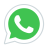# MATRICES AND DETERMINANT

Hello Dear, In this Course you will learn basic concept of Matrix, Some types of matrices like equal matrix square matrix rectangular matrix, when two matrices are called equal matrices, how we can id...
created by Ali Raza
Last updated 2021-06-29
Enrollment: 2
Language: Urdu
\$5
\$25
This course has
Access on mobile
Certificate of Completion

• Basic Concept of Matrices
• Types of Matrices
• Matrices Operation
• Determinant
• Solution of System of Linear equation

1. none

## Which Students must take this course

1. Matric, Intermediate, BSc

## Course Content

Introduction to Course
00:05:54
Matrix , Rows , Column , Equal Matrices
00:20:02
Types of Matrices
00:13:07
Multiplicative Inverse
00:15:04
Solution of System of linear equations
00:13:56

## Description

Hello Dear, In this Course you will learn basic concept of Matrix, Some types of matrices like equal matrix square matrix rectangular matrix, when two matrices are called equal matrices, how we can identify that a given matrix is diagonal scalar or identity matrix, how we can find the transpose of a matrix, which matrix is called null or zero matrix, how we can find negative of a matrix, difference between symmetric matrix and skew symmetric matrix, some matrix operations like addition, subtraction and multiplication, how we can find the determinant of a 2 by 2 matrix, how we can identify that a given matrix is singular or non singular, how to find the adjoint of a matrix, how we can find the multiplicative inverse of a matrix, and finally and very important topic The solution of system of linear equations using CRAMER's rule and Matrix Inversion Method... for more detail please watch my course intro video.... THNX

Message 1 sec ago
URL has been copied